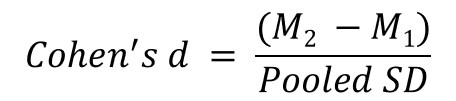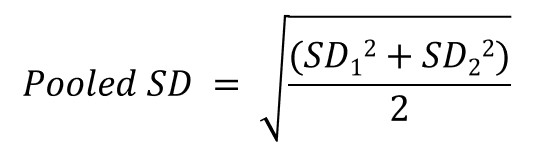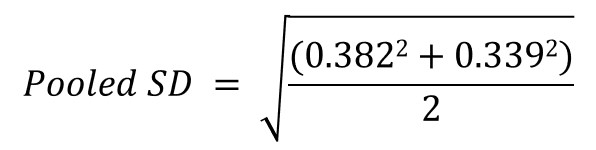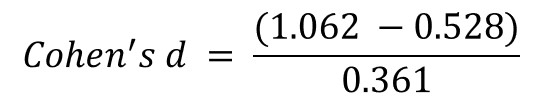What is Cohen’s d?

Cohen’s d is a type of effect size between two means. An effect size is a quantitative measure of the magnitude for the difference between two means, in this regard. Cohen’s d values are also known as the standardised mean difference (SMD).

Since the values are standardised, it is possible to compare values between different variables. Further, the use of d values is often applied in meta-analyses to compute pooled effect sizes of different studies.

What is the Cohen’s d formula?

The Cohen’s d formula is actually really simple. Specifically, it is the difference between two means and divided by the pooled standard deviation. The formula for Cohen’s d can be seen below.To calculate the pooled SD, the equation is displayed below. But don’t fear, it is not as bad as you may think.The components of the formulas are:

• M1 = The mean of group 1 (e.g. control group)
• M2 = The mean of group 2 (e.g. experimental group)
• SD1 = The standard deviation of group 1
• SD2 = The standard deviation of group 2

Calculating Cohen’s d by using an example

Now you know the formula, let’s use it in an example.

We want to calculate the Cohen’s d between two groups: male and females. Specifically, a certain protein was quantified in the blood of the two groups. Females had higher levels of the protein (1.062 ± 0.339) than males (0.528 ± 0.382).

Therefore, the four components for the equation are:

• M1 = 0.528
• M2 = 1.062
• SD1 = 0.382
• SD2 = 0.339

The easiest way to calculate d values is to firstly calculate the pooled standard deviation. Thus, taking these values and entering them into the equation are shown below.Doing so will give a pooled standard deviation value of 0.361. So, now we can put this value into the Cohen’s d equation along with the two group means. The full equation is displayed below.Plugging all of that into a calculator will give a d value of 1.479.

But what does the d value actually represent? In the next section, I will explain what the values stand for and how to interpret them.

The Cohen’s d online calculator

If you are still struggling to calculate d values by using the formula, we have created a Cohen’s d calculator.

To use the calculator, simply enter the group mean and standard deviation values, and the d effect size will be calculated for you.

How to interpret Cohen’s d effect sizes

Simply, you can think of Cohen’s d values as standard deviations between the two groups.

A value of 1 indicates that the means of the two groups differ by 1 standard deviation. Taking our example from before, a value of 1.479 indicates the mean differences between the male and female groups differ by 1.479 standard deviations, which is quite a large effect as you will see.

What are small, medium and large effect sizes?

After calculating d values, people often state if the effect size is either: small, medium or large. Cohen himself provided a guide for what values should indicate which size in his book. These are presented below. However, the terms may be dependent upon your field of study.

Small: d = 0.2

Small effect sizes are considered too small to be differentiated by the naked eye. Cohen gave the example of a small effect size as, the difference in height between 15- and 16-year-old girls.

Medium: d = 0.5

Medium effect sizes are just larger enough to be seen by the naked eye. Elaborating on this, Cohen explained that the difference in height between 14- and 18-year-old girls would be calculated as a medium effect size.

Large: d = 0.8

Large effect sizes are really obvious differences between groups. Keeping in alignment with Cohen’s examples, he described this size to be observed when comparing 13- and 18-year-old girl heights.